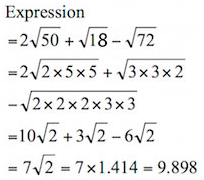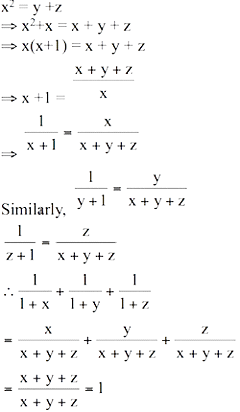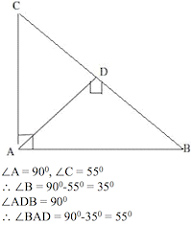# Sample Aptitude Questions of EXL

1. The simple interest on Rs. 4000 in 3 years at the rate of x% per annum equals the simple interest on Rs. 5000 at the rate of 12% per annum in 2 years. The value of x is
1. 8%
2. 9%
3. 10%
4. 6%
Answer: Option C.
S.I = Principal*Time*Rate/100
Therefore 4000*3**/100 = 5000*2*12/100 => * 5*2*12/4*3
=10% per annum
1.  If x2 – 3x + 1 = 0, then the value of x2 + x + 1/x + 1/x2 is
1. 6
2. 8
3. 10
4. 2
Answer: Option C.
x2 - 3x + 1 = 0
=> X2 + 1 - 3x
Dividing both sides by x, => X + 1/3 - 3
Therefore x2 + x + 1/x + 1/x2
= (x2 + 1/x2) + (x + 1/x)
= (x + 1/x)2 -2+ (x + 1/x)
= 9-2 + 3 = 10
2. If (4x - 3)/x + (4y - 3)/y + (4z - 3)/z = 0, then the value of 1/x + 1/y + 1/z is
1. 4
2. 6
3. 9
4. 3
Answer: Option A.
Z(4x - 3)/x + (4y - 3)/y + (4z - 3)/z = 0
=> 4x/x - 3/x + 4y/y - 3/y + 4z/z - 3/z = 0
=> 3/x + 3/y + 3/z = 4 + 4 + 4 = 12
=> 1/x + 1/y + 1/z = 12/3 = 4
3. Find the simplest value of 2√50 + √18 - √72 (given √2 = 1.414)
1. 10.312
2. 8.484
3. 4.242
4. 9.898
Answer: Option D.4. If a2 + b2 + 4c2 = 2(a + b – 2c) – 3 and a, b, c are real, then the value of (a2 + b2 + c2) is
1. 2
2. 21/4
3. 3
4. 31/4
Answer: Option B.
a2 + b2 + 4c2 = 2a + 2b - 4c-3
=> a2 + b2 + 4c2 - 2a - 2b + 4c + 1 = 0
=> a2 - 2a + 1 + b2 - 2b + 1 + 4c2 + 4c + 1 = 0
=> (a-1)2 + (b-1)2 + (2c+1)2 = 0
Therefore a-1 = 0 => a = 1;
b-1 = 0 => b = 1;
2c+1 = 0
=> c = - 1/2
Therefore a2 + b2 + c2 = 1 + 1 + 1/4
= 2 1/4
5. A number x when divided by 289 leaves 18 as a remainder. The same number when divided by 17 leaves y as a remainder. The value of y is
1. 3
2. 1
3. 5
4. 2
Answer: Option B.
Here, the first divisor (289) is a multiple of second divisor (17)
∴ Required remainder = Remainder obtained on dividing 18 by 17 = 1. Hence the answer is option 2.
6. An equation of the form ax + by + c = 0 where a ≠ 0, b ≠ 0, c = 0 represents a straight line which passes through
1. (0, 0)
2. (3, 2)
3. (2, 4)
4. None of these
Answer: Option A.
Ax+by+c = 0
When c = 0
ax+by = 0
by = -ax ⇒ y = - ax/b
when x = 0, y = 0 i.e., this line passes through the origin (0,0).
7. The numerator of a fraction is 4 less than its denominator. If the numerator is decreased by 2 and the denominator is increased by 1, then the denominator becomes eight times the numerator. Find the fraction.
1. -4/8
2. 2/7
3. 3/8
4. 3/7
Answer: Option D
Original fraction = (x - 4)/x
In case II,
8(x - 4 - 2) = x + 1
⇒ 8x - 48 = x + 1
⇒ 7x = 49 ⇒ x = 7
∴Original fraction
= (7 - 4)/7 = 3/7
8. If x2 = y + z, y2 = z + x and z2 = x + y then the value of 1/(1+x) + 1/(1+y) + 1/(1+z) is
1. 2
2. 0
3. -1
4. 1
Answer: Option D.9. In a triangle ABC, ∠A = 90°, ∠C = 55°, AD bar ⊥ BC bar. What is the value of ∠BAD?
1. 45o
2. 55o
3. 35o
4. 60o
Answer: Option B.